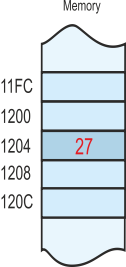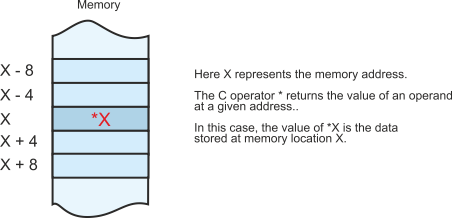Two of the most common terms in computer science are address and data. Data is the term used to describe information, and address is the term used to describe the location of an item of information.  It all sounds very simple and obvious; but it is anything but simple. Fellow professors tell me that some of their students find the distinction between an address and data difficult, an observation I’ve long noticed.

So, why are the concepts of address and data difficult to comprehend? After all, I’m Alan Clements and I live at 12 Merrington Avenue, so how could anyone confuse me with my address? The distinction is obvious in the non-computer world,  but the distinction between address and data in computing can be rather subtle. Consider the following fragment of C language that uses addresses and data.

int t, *b;

int a;

b = &a;

t = *b;

Here the operators * and & are used to deal with addresses. In this case, the distinction between address and data are not so immediately obvious to the novice now.

Consider the following operation in a low-level language (expressed both in ARM assembler and 68K assembler):

LDR   r1,[r1]      ;ARM assembler

MOVE  (A1),A1      ;68K assembler

Both instructions perform the same action. They take a pointer to data, and replace the pointer with that data Once again, here’s an operation involving the concepts of data and addresses and yet the implications of this operation are not immediately blindingly obvious someone encountering these concepts for the first time.

Data

Defining the term data is difficult because it is used in different ways by different people. In computer science, data generally refers to information stored in a computer in binary form. Data may be a single element (e.g., a number or a letter of the alphabet) or it may be a collection of elements such as an array, a list, a string, or a table.

Although data elements can be represented in many different ways, computer designers have limited the range of options for practical reasons; that is, data comes in units of  8 bits (the byte), 16 bits (the half word), 32 bits (the word), and 64 bits (the double word). Note that some computer architectures use these terms differently. They have not been standardized.

The type of data represented is normally restricted to integer, real (floating-point), or character. These restrictions are used simply because of the way in which computer instructions operate on data.

In everyday life, an address represents the location of a person or building. In computer science it is used to represent the location of an item of data in memory. A computer memory can be regarded as a very large array of storage elements. Each of these memory storage elements may  hold an item of data; for example, you might say that memory location 0x12345678 holds the integer value 0x57 (the 0x prefix is used because I am expressing addresses and data as hexadecimal values).

x = x + 1

that is, we increment the value of data element x by 1.

This is not quite as simple as might appear. First, we’ve abandoned the rules of algebra that we learned at high school. The expression x = x + 1 looks like an equation but it isn’t. We really should have used a notation like

x x + 1

to indicate that the value on the left is replaced by the value on the right. Some languages do indeed do this and require the use of a special symbol like =: to indicate a transfer of data.

There’s another problem. What exactly is x? This goes to the heart of the address/data confusion. When we write x we are using it to indicate a data value that is stored somewhere in memory.

So, x is really the address of a data element. When we see x in the equation we automatically think of the value of x and not its address; in other words we mentally convert the address x into the corresponding data at a subconscious level without thinking about it.

Unfortunately, when writing programs we have to be acutely aware of the distinction between x the address and x the data stored at that address.

Memory, data and C

Let’s look at how data is stored in memory and how the C language represents addresses and data. The following figure illustrates a memory map with actual numeric addresses and data. In general, no one works with numeric data like this because we don’t care where data lives in memory. Only computer architects are interested at this level because their role is to design computer systems.

We are going to assumes a 32-bit word of 4 bytes. If the computer is byte-addressed (i.e., each and every byte has a unique address) then consecutive memory words differ by 4 bytes; that is, 0000, 0004, 0008, 000C, 0010, 0014,  and so on. Remember that addresses are given in hexadecimal form.

In this example, memory location 0x1204 contains the value 0x27. The numerical value 0x1204 is the address of the data, and the numerical value 0x27 is the actual data.

We can write p = [q] to indicate that p is the value of the data at the address q. The square brackets are read as “the contents of”, so that p = [q] can be read as “p is the contents of memory location q”.

The next figure is effectively the same as the previous one except that we have used the symbolic name X to represent the location of a variable. Here’s where confusion can creep in. If you ask what’s the value of X you can get two answers. If you mean “what is the address represented by X”, then the answer is 0x1204. If you mean “what is the data at the address represented by X”, the answer is 0x27.

These two concepts can be represented algebraically as X = 0x1204 (X is the address 0x1204) or as 0x27 = [X] (the data stored in X is 0x27).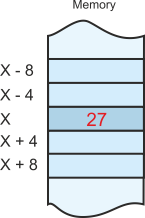C is a high-level language that makes the distinction between address and data explicit. Indeed, C is sometimes called a high-level assembly language. The next figure shows how C uses the * operator to return the value of the data at a given address.

If X is an address, then *X is the contents of that address. In other words, the * operator is equivalent to the use of [ ] in RTL (register transfer language) notation.

The next figure illustrates C’s & operation, which is the inverse of *. The & operator returns the address of a variable. In this case, if the variable is X, then &X is the address of that variable.

If you think about it, and it’s rather best not to, the value of *&X is X.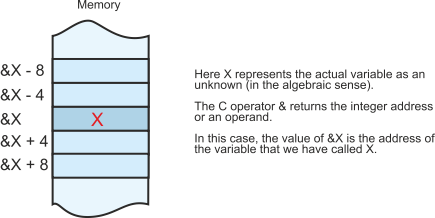Address and data in ARM assembly language

Apart from some special block move instructions, the ARM provides only two instructions that access memory. These are a load a register with data from memory instruction and a store the data in a register somewhere in memory. These are complementary operations.

Consider first the load register instruction, LDR. You might expect to see a LDR r1,0x12345678 instruction whose purpose is to copy the contents of memory location 0x12345678 into register r1. Such an ARM instruction does not exist simply because there is no room in an op-code for the memory address.

The format of the ARM’s load instruction is LDR r1,[r2]. Instead of specifying the actual address of the operand, we’ve specified where the address is. In this case the address is the contents of register r2. In RTL terms we have

[r1]  [[r2]]

This expression is read as, “Load register r1 with the contents of the memory locations whose address is specified by the contents of register r2”.

The address of the operand is called an indirect address or a register indirect address, or a pointer-based address. All of these terms indicate that accessing a data element is a two-step process. First the register containing the address is read and then the memory location containing the required data is read. The following figure illustrates this concept.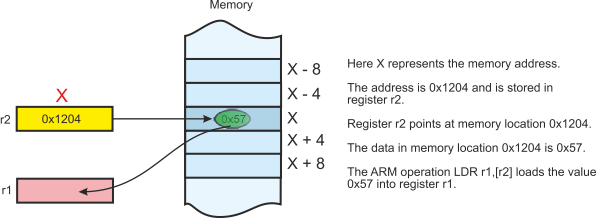Pointer register r2 contains the address of the variable. The variable is called X and its address is 0x1204. At memory location 0x1204 there is a data element 0x57. If we execute LDR r1,[r2] we load register r1 with the value 0x57. We do not load register r1 with 0x1204.

Back to X = X + 1

If we wish to carry out this operation we can write

LDR r1,[r2]     ;load X into register r1

STR r1,[r2]     ;store X in memory

Note how the machine level code requires us to understand address and data, whereas the comments use plain English to describe what’s going on and the distinction is less clear.

We haven’t stated how the address X gets in register r1 or  how the data value X gets in memory location 0x1234. Assume that before the above code is run we have executed something like.

MOV r0,#0x57    ;load the actual value of X into register r0

STR r0,[r2]     ;store X in memory

Now we’ve set up the value of X in memory and the address of X in register r2.

Using Symbolic names

In a real program, you don’t want to worry about actual addresses. Let the computer sort that our automatically. Using the Keil Arm assemble we can write

ENTRY

ADR r2,X        ;register r2 points to X

MOV r0,#0x57    ;register r0 contains the value of X

STR r0,[r2]     ;initialize X in memory

;now we can do the X + 1

LDR r1,[r2]     ;load X into register r1